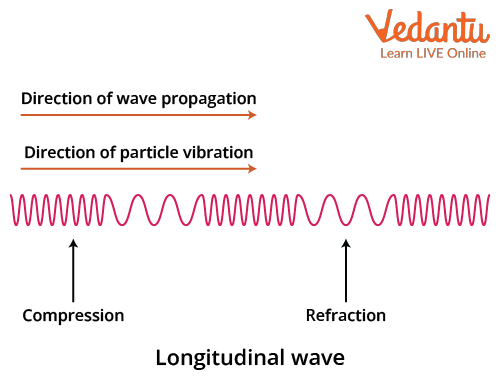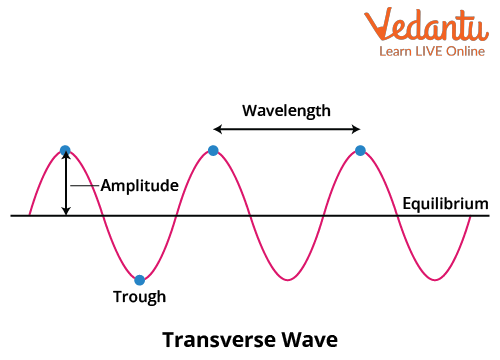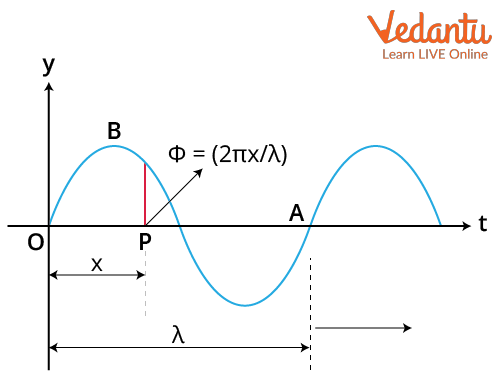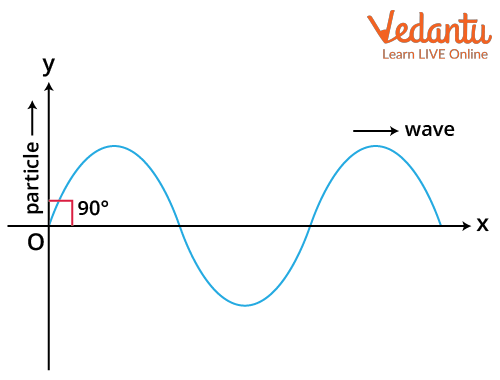Courses
Courses for Kids
Free study material
Free LIVE classes
More

# Particle Velocity | Correlation between Particle and Wave Velocity for JEE## A Brief Introduction to the Topic

Last updated date: 24th Mar 2023
Total views: 80.4k
Views today: 0.57k

When applied to a sound wave across a medium of air, particle velocity would be the physical speed of a very small amount of fluid distinguished throughout its dynamic history, as it moves alternatingly in the direction of the sound wave. Particle velocity is not the equivalent to the speed of sound. The wave proceeds comparatively fast, while the particles oscillate in all sides of their original position with a relatively small particle velocity.

## What is Particle Velocity?

The particles or molecules of a medium are replaced from their arbitrary motion in the existence of a sound wave and developed oscillation by the frequency correlated with the wave. The speed of the particle throughout displacement is known as the particle velocity, and its connections to the sound pressure p is specified by the following relation:

$p=\rho\cdot c\cdot u$

Where, $\rho=$ density of the medium, c= sound velocity, u= particle velocity, which is the root mean square of the expeditious particle velocities over a time interlude at the given position.

The amplitude of the displacement d is associated with the particle velocity by the equation:

$u=\dfrac{d}{2 \pi \mathrm{f}}$

where f= frequency of the wave.

## Particle Velocity Formula

For a given wave equation,

$y=A \sin (\omega t - kx)$

The particle velocity can be determined by doing the simple derivative of the above equation with respect to time t . So we get:

$\dfrac{d y}{d t}=A \omega \cos (\omega t - kx)$

So the particle velocity will be:

$v_{p}=A \omega \cos (\omega t - kx)$

## What is Wave Velocity?

Wave velocity refers to the distance crossed by a periodic or cyclic motion per unit time in any orientation. Wave velocity infers both speed and direction. The velocity of a wave is equal to the product of its wavelength and frequency (number of vibrations per second). Also, the wave velocity is individualistic of its intensity. The wave velocity relies upon the nature of the medium used. This is also known as the phase velocity.

Mathematically, the formula of wave velocity is:

$v=\dfrac{\omega}{k}$

Where, $\omega =$angular velocity, k = the angular wave number or propagation constant.

It can be expressed by the equation as:

$k=\dfrac{2\pi}{\lambda}$

Where $\lambda$ is the wavelength of the promulgating wave.

## How Particles are Transferred in Longitudinal Wave?

In longitudinal waves, the particles transfer energy from one position to another by vibrating in the line of orientation of the wave promulgation. The motion of  the particles is usually parallel to the movement of energy. Here, the particles are also overridden parallel to the promulgating wave orientation. An example of longitudinal waves is ultrasonic waves:Propagation of Longitudinal Wave

## Movement of the Particles in Transverse Wave

In transverse waves, the  movement of the particles is at right angles to the movement of the promulgating waves. In a transverse wave, the particles are overridden perpendicular to the orientation to the wave which is propagating. Examples of transverse waves incorporate vibrations on a guitar string. Other examples are radioactive waves, electromagnetic waves etc.Propagation of Transverse Wave

## Correlation between Particle and Wave Velocity in Plane Progressive Wave

### What is Plane Progressive Wave?

A plane progressive congruous wave is a wave which progresses in given directions without changing its conformation. Every particle of medium accomplishes simple congruous motion about their mean position with equal amplitude and time period.

### Derivation of the CorrelationPlane Progressive Wave

As the equation of a plane progressive wave is:

$y=A \sin (\omega t-k x)$.....(i)

So particle velocity will be:

$v_{p}=\dfrac{d y}{d t}=A \omega \cos (\omega t-k x)$.......(ii)

The slope of the displacement curve or strain is:

$\dfrac{d y}{d x}=-A k \cos (\omega t-k x)$........(iii)

Now, by dividing equation no. (ii) by equation no. (iii) we will get:

\begin{align} &\dfrac{\dfrac{d y}{d t}}{-\dfrac{d y}{d x}}=\dfrac{\omega}{k} \\ &\Rightarrow \dfrac{v_{p}}{-\dfrac{d y}{d x}}=v \quad\quad\left(\text{since}, \dfrac{\omega}{k}=v, \text { wave velocity }\right)\\ &\Rightarrow v_{p}=-v \dfrac{d y}{d x} \end{align}

So we can conclude that the particle velocity is equal to the product of wave velocity and strain.

## Angle between Particle Velocity and Wave Velocity

In a transverse wave, the particle of the medium vibrates equilatera (perpendicular) to the direction in which the wave promulgates. Hence the angle between these two is: $\dfrac{\pi}{2}=90^{\circ}$Transverse Wave

## Speed of Particle

Speed of a particle is defined as the absolute value of the particle velocity. That means that the speed of the particle is equal to the modulus of the velocity of the particle which can be understood by the following equation:

$\text { speed of the particle}=\left|v_{p}\right|$

If the velocity of the particle is -5 $\dfrac{m}{s}$ then the speed will become 5 $\dfrac{m}{s}$.

## Conclusion

The particle velocity is a function of time which can be varied with the variation of time but on the other hand the wave velocity is independent of time. It is considered as constant for a certain medium. The wave velocity can only be changed when the property of the medium through which it travels is changed.

In the progressive or transverse wave, the particles move faster than the wave because the particle velocity depends on the multiplication product of wave velocity and the strain. This article covers all the important information about the topic including the relation and angle between the particle and wave velocity in a progressive wave.

## FAQs on Particle Velocity | Correlation between Particle and Wave Velocity for JEE

1. Mention the different kinds of waves.

Waves are basically of two types: longitudinal and transverse. Longitudinal waves are the waves where the displacement of the medium is in the same orientation as that of the orientation of the itinerant wave. Flexible waves such as those in water, surface up and down, and longitudinal waves similar to those of sound, combine altering pressure and abnormal occurrences.

As per scientific experiments, electromagnetic waves are classified into seven basic types:

2. Microwaves

3. Visible Light Rays

4. Ultraviolet Waves

5. Infrared Waves

6. Gamma Rays

7. X-Rays

2. What is particle acceleration?

Acceleration of particles (electrons, protons and other charged particles) is obtained by projecting them with electromagnetic waves. The energy of the waves is transferred to the particles as the particles progress through special cavities made of copper or superconducting material. Acceleration is explained technically as "the rate of change of velocity of an object with respect to time". It can be expressed by the following equation:

$a=\dfrac{d v}{d t}$

Where,  a is the acceleration vector and v  is the velocity vector expressed in $\dfrac{m}{s}$ (SI unit).

3. What are the features of the oscillation of waves?

The oscillating waves recline perpendicular to the orientation where oscillation takes place. The waves transfer energy instead of moving in the direction of propagation. Oscillation factors such as output power, oscillation frequency and operating temperature improve rapidly. Apart from this, direct excretion from the oscillators as well as high frequency transformation from low frequencies with recurring amplifiers can provide sub-terahertz emissions. Repetitive movements are called periodic movements. The object in such a movement is moving about the equilibrium position due to the reversal force or torque.